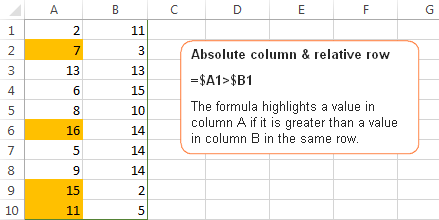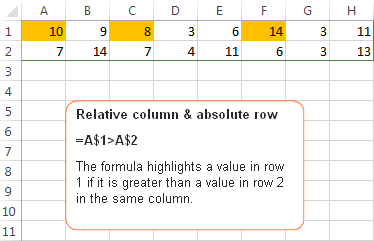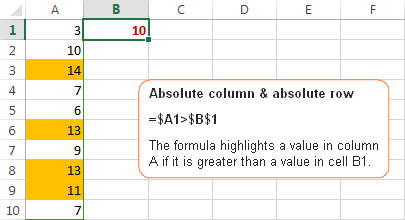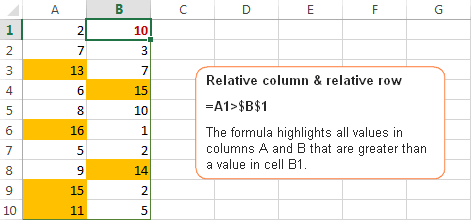# Relative and absolute cell references in Excel conditional formatting rules

Recently we have published a few articles covering different aspects of Excel conditional formatting. Unexpectedly, it's turned out that it's not creating a rule and even not making a proper formula that represents the greatest challenge. Using proper cell references in Excel formulas appear to be the most complex part and a common source of problems.

"I had my conditional rule correct, except for the mixed references." This is what our blog readers have often reported in comments. So, why don't we invest a few minutes to figure this thing out? This will certainly save you far more time in the long run!

## How relative and absolute cell references work in conditional formatting rules

In all Excel formulas, including conditional formatting rules, cell references can be of the following types:

• Absolute cell references (with the \$ sign, e.g. \$A\$1) always remain constant, no matter where they are copied.
• Relative cells references (without the \$ sign, e.g. A1) change based on the relative position of rows and columns, when copied across multiple cells.
• Mixed cells references (absolute column and relative row (e.g. \$A1), or relative column and absolute row (e.g. A\$1). In Excel conditional formatting rules, mixed cell references are used most often, indicating that a column letter or row number is to remain fixed when the rule is applied to all other cells in the selected range.

In conditional formatting rules, cell references are relative to the top-left most cell in the applied range. So, when making a new rule, you can simply pretend as if you are writing a formula for the top-left cell only, and Excel will "copy" your formula to all other cells in the selected range.

Now, let me show you a few examples that demonstrate how seemingly identical formulas produce different results depending on what cell references types are used.

## Example 1. Absolute column and relative row

This pattern is most typical for conditional formatting rules and in 90% of cases cell references in your Excel conditional formatting rules will be of this type.

Let's make a very simple rule that compares values in columns A and B and highlights a value in column A if it is greater than a value in column B in the same row.

If you need the detailed instructions on how to create conditional formatting rules with formulas, here you go - Creating an Excel conditional formatting rule using a formula. In this case, the formula is obvious:

`=\$A1>\$B1`

Because you always compare values in columns A and B, you "fix" these column by using absolute column references, notice the \$ sign before the column letters in the above formula. And, since you are comparing the values in each row individually, you use relative row references, without \$.## Example 2. Relative column and absolute row

This cell reference type is the opposite of the previous one. In this case, the row number is always constant while the column changes. You use such references when you want to check values in a given row against a certain value or against values in another row.

For example, the below formula compares values in row 1 and 2 and the rule highlights a value in row 1 if it is greater than a value in row 2 in the same column:

`=A\$1>A\$2`Because you want the row numbers to be fixed, you use the absolute row references, with the \$ sign. And, because you want to compare values in each column individually, you create the rule for the left-most column (A) and use relative column references, without the \$ sign.

## Example 3. Absolute column and absolute row

You use absolute row and absolute column references if you want to compare all values in the selected range with some other value.

For example, let's create a rule that highlights all values in column A that are greater than a value in cell B1. The formula is as follows:

`=\$A1>\$B\$1`

Please pay attention to the use of the following references:

• \$A1 - you use an absolute column and relative row references because we want to check values in all cells of column A against the value in cell B1.
• \$B\$1 - you use absolute column & absolute row because cell B1 contains the value you want to compare all other values against and you want this cell reference to be constant.## Example 4. Relative column and relative row

This reference type is used in Excel conditional formatting rules least of all. You use relative column & relative row references when you want to check all cells of the selected range against a certain value.

Suppose, you want to highlight all cells in columns A and B that are greater than a value in cell B1. You can simply copy the formula from the previous example and replace \$A1 with A1 since you do not want to fix either row or column:

`=\$A1>\$B\$1`

Remember, you write the formula for the top-left cell in your range, A1 in our case. When you create a rule with the above formula and apply it to some range, say A1:B10, the result will look similar to this:Tip. To quickly toggle between absolute and relative references, select the cell reference in the formula bar and press the F4 function key. The reference will rotate between the four types from relative to absolute, like this: A1 > \$A\$1 > A\$1 > \$A1, and then back to the relative reference A1.

## Useful resources

Hopefully, these simples examples have helped you fathom out the essence of relative and absolute cell references in Excel. Now that you know how to determine the appropriate reference type for your rules, go ahead and harvest the power of Excel conditional formatting for your projects. The following resources may prove helpful:War in Ukraine! To support Ukraine and save lives please visit this page.